# Use your data to estimate Ka of the weak acid. Show all working (give your answer...

Use your data to estimate Ka of the weak acid. Show all working (give your answer to thee sig figs)

pH at Equivalence point=7.5

volume of base added at equivalence point (to two decimal places)=12.10

This Homework Help Question: "Use your data to estimate Ka of the weak acid. Show all working (give your answer..." No answers yet.

We need 10 more requests to produce the answer to this homework help question. Share with your friends to get the answer faster!

0 /10 have requested the answer to this homework help question.

Once 10 people have made a request, the answer to this question will be available in 1-2 days.
All students who have requested the answer will be notified once they are available.
Similar Homework Help Questions
• ### pH at equivalence is 8.40 It's a tritration with a breaker filled with 0.1894 M CH3COOH...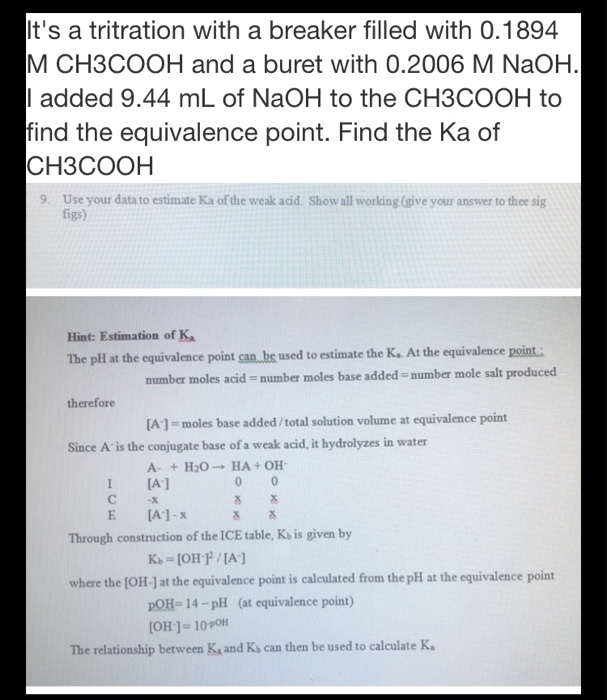pH at equivalence is 8.40 It's a tritration with a breaker filled with 0.1894 M CH3COOH and a buret with 0.2006 M NaOH. | added 9.44 mL of NaOH to the CH3COOH to find the equivalence point. Find the Ka of CH3COOH 9. Use your data to estimate Ka of the weak acid. Show all working (give your answer to thee sig figs) Hint: Estimation of K The pH at the equivalence point can be used to estimate the K....

• ### Titration of Weak Acid with Strong Base A certain weak acid, HA, with a Ka Value of 5.61 *10^-6, is titrated wit...

Titration of Weak Acid with Strong Base A certain weak acid, HA, with a Ka Value of 5.61 *10^-6, is titrated with NaOH. PART A A solution is made by mixing 8.00 mmol(millimoles) of HA and 1.00 mmol of the strong base. What is the resulting pH? express the pH numerically to two decimal places. pH = ? PART B More strong base is added until the equicalence point is reached. What is the pH of this solution at the...

• ### A certain weak acid, HA, with a Ka value of 5.61×10−6, is titrated with NaOH. ------------------------------------------------------------...

A certain weak acid, HA, with a Ka value of 5.61×10−6, is titrated with NaOH. ------------------------------------------------------------ A. A solution is made by titrating 8.00 mmol (millimoles) of HA and 2.00 mmol of the strong base. What is the resulting pH? Express the pH numerically to two decimal places. B. More strong base is added until the equivalence point is reached. What is the pH of this solution at the equivalence point if the total volume is 43.0 mL ? Express...

• ### A student peforms a titration, titrating 25.00 mL of a weak monoprotic acid, HA, with a...

A student peforms a titration, titrating 25.00 mL of a weak monoprotic acid, HA, with a 1.22 M solution of NaOH. They collect data, plot a titration curve and determine the values given in the below table. ml NaOH added pH Half-way Point 18.34 4.06 Equivalence point 36.68 8.84 How many moles of NaOH have been added at the equivalence point? mol What is the total volume of the solution at the equivalence point? mL During the titration the following...

• ### Based on the following information... please answer A. B. and C. TITRATION OF A WEAK ACID...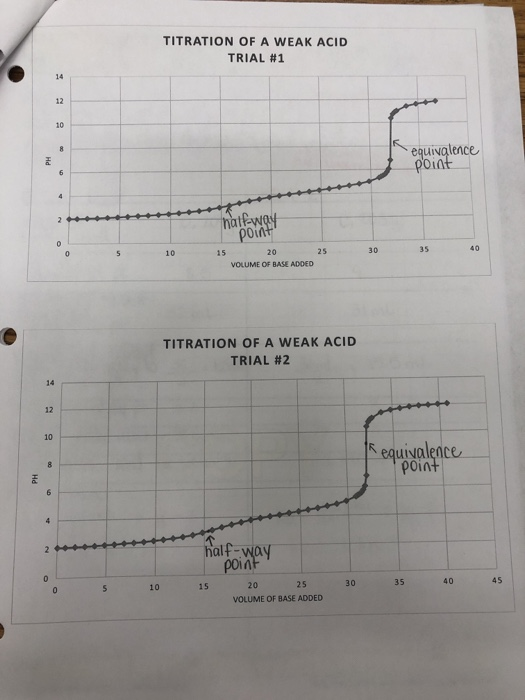Based on the following information... please answer A. B. and C. TITRATION OF A WEAK ACID TRIAL #1 equivalence point halfway point 25 VOLUME OF BASE ADDED 30 35 40 TITRATION OF A WEAK ACID TRIAL #2 1 equivalence point Hd half-way point s 10 15 30 35 40 45 20 25 VOLUME OF BASE ADDED Trial 1 Trial 2 Mass of oxalic acid 0.2039 pH at equivalence point a Mass of oxalic acid 0.2099 at equivalence point nt 8,5...

• ### Titration of weak acid with strong base pre-lab Answers can only be entered/graded between 2019-10-24 and...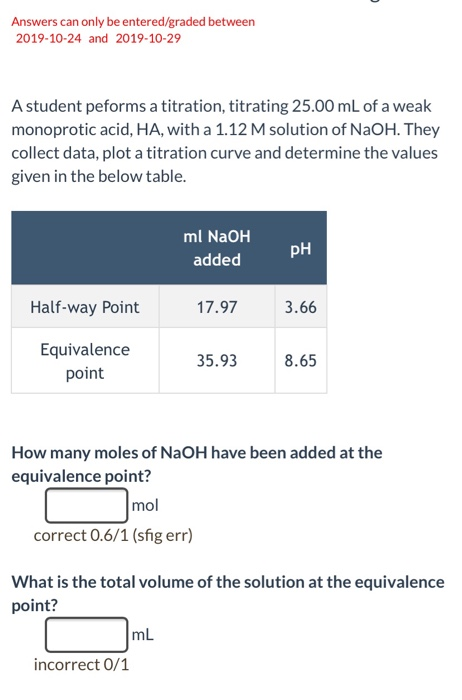Titration of weak acid with strong base pre-lab Answers can only be entered/graded between 2019-10-24 and 2019-10-29 A student peforms a titration, titrating 25.00 mL of a weak monoprotic acid, HA, with a 1.12 M solution of NaOH. They collect data, plot a titration curve and determine the values given in the below table. ml NaOH PH added Half-way Point 17.97 3.66 Equivalence point 35.938.65 How many moles of NaOH have been added at the equivalence point? r mol correct...

• ### 3.)A certain weak acid, HA, with a Ka value of 5.61×10?6, is titrated with NaOH. Part A A solution is made by titrati...

3.)A certain weak acid, HA, with a Ka value of 5.61×10?6, is titrated with NaOH. Part A A solution is made by titrating 7.00 mmol (millimoles) of HA and 1.00 mmol of the strong base. What is the resulting pH? Express the pH numerically to two decimal places. Part B More strong base is added until the equivalence point is reached. What is the pH of this solution at the equivalence point if the total volume is 35.0 mL ?...

• ### A student peforms a titration, titrating 25.00 mL of a weak monoprotic acid, HA, with a...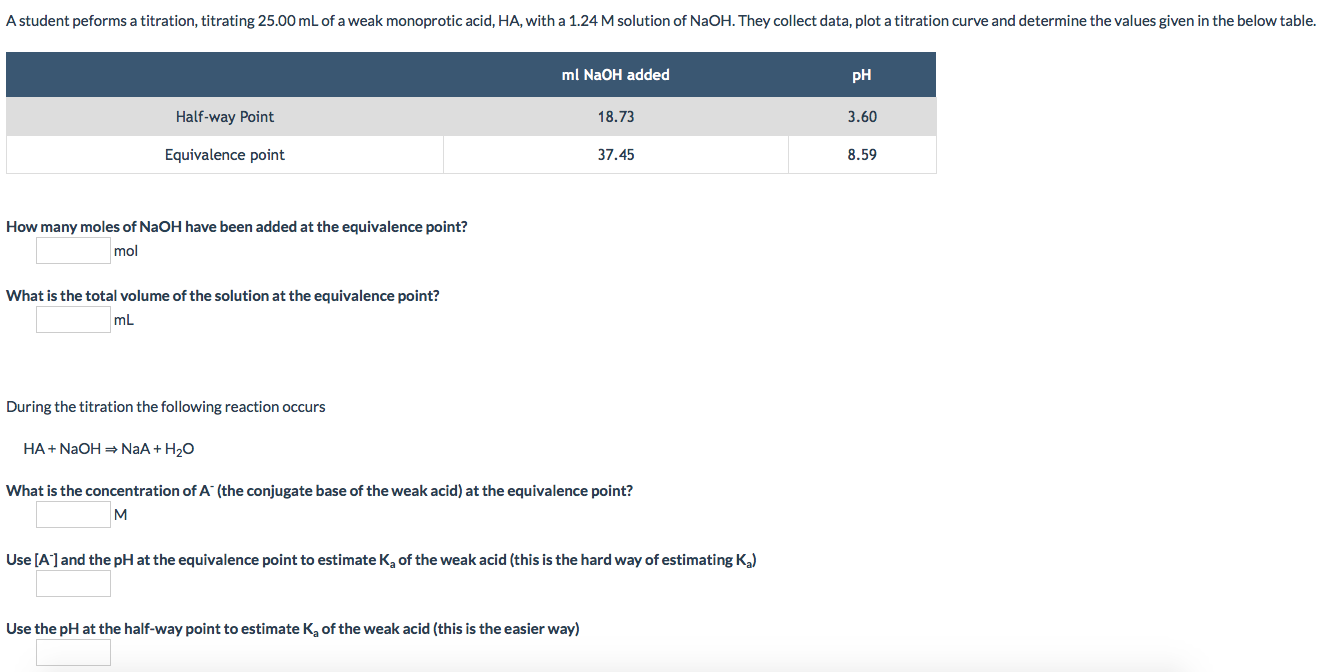A student peforms a titration, titrating 25.00 mL of a weak monoprotic acid, HA, with a 1.24 M solution of NaOH. They collect data, plot a titration curve and determine the values given in the below table. ml NaOH added pH Half-way Point 18.73 3.60 Equivalence point 37.45 8.59 How many moles of NaOH have been added at the equivalence point? mol What is the total volume of the solution at the equivalence point? mL During the titration the following...

• ### A student peforms a titration, titrating 25.00 mL of a weak monoprotic acid, HA, with a...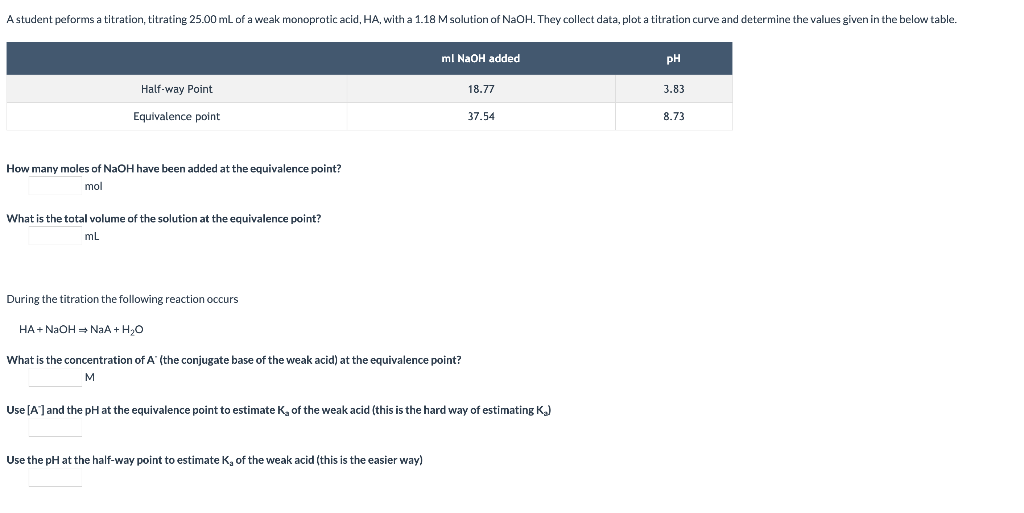A student peforms a titration, titrating 25.00 mL of a weak monoprotic acid, HA, with a 1.18 M solution of NaOH. They collect data, plot a titration curve and determine the values given in the below table. ml NaOH added pH Half-way Point 18.77 3.83 Equivalence point 37.54 8.73 How many moles of NaOH have been added at the equivalence point? mol What is the total volume of the solution at the equivalence point? mL During the titration the following...

• ### Tuo UF Weak Acid with Strong Base 5 of 7 > A certain weak acid, HA,...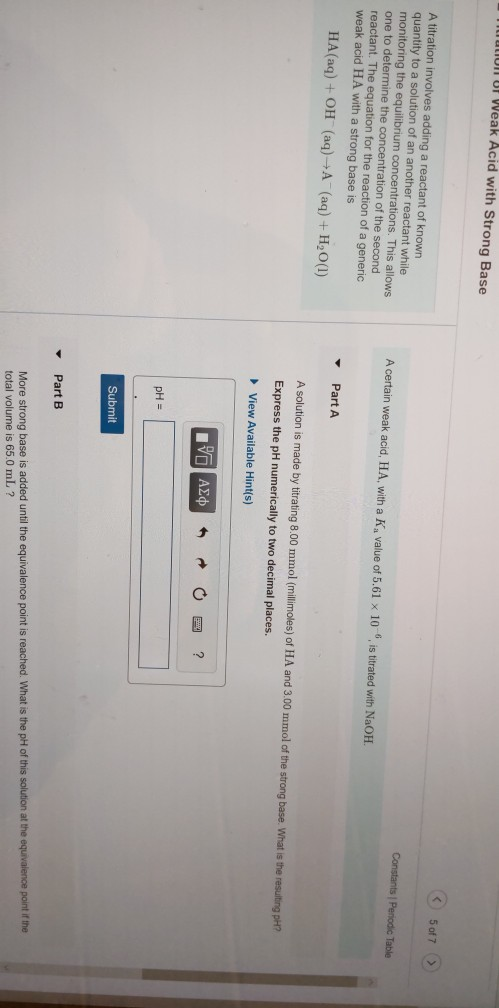Tuo UF Weak Acid with Strong Base 5 of 7 > A certain weak acid, HA, with a Ka value of 5.61 x 10 Constants Periodic Table A titration involves adding a reactant of known quantity to a solution of an another reactant while monitoring the equilibrium concentrations. This allows one to determine the concentration of the second reactant. The equation for the reaction of a generic weak acid HA with a strong base is HA(aq) + OH (aq) +...

Free Homework App